# Interior Sketchbook Diary

This sketchbook is filled with images based off of the famous mandelbrot iterator z = z2 + c where z and c are complex. All of these sketches use c as the coordinate plane, and show various relationships between c and z in color.

Additional explorations of the interior can be found in the M-Set Derivations Room and the Spectral Analysis Room.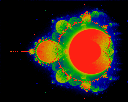Hausdorf measure of Mandelbrot Interior. This picture is created by dividing up the complex plane into pixels, and counting how many times each pixel is visited as the iterator is iterated. Red pixels are visited often; yellow, green, blue and black, respectively, less so. The red outline shows boundary of set, as usually seen.Lyapunov Exponent of iterates. Red indicates a large positive (divergent) exponent, moving through yellow, green and blue to black, which indicates a small or negative (attractive) exponent.Lyapunov Exponent of iterates, this time showing the attractive strength. Red shows regions of strong attraction. Note that it appears that some of the attractors lie outside the Mandelbrot set. Note that these attractors seem to correspond to zeros of iterates of various orders. (See below).

It is very clear from these pictures that the orbits that the iterates take can take then far outside the "basin of attraction" of the mandelbrot set. This is not a contradiction: the orbit of a point is in no way constrained to lie within the basin of attraction. Less obvious is whether the limiting orbit (limit cycle) must lie entirely within the basin of attraction: after all a "basin" is a statement about initial values, while limit cycles are a statement about final values. In fact, the third graphic appears to show that some areas of attractive Lyapunov exponent lie outside of the basin of attraction. These points are very tiny, and could, of course, be due to the numerical methods used, or they may be saddle points.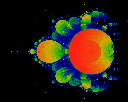Hausdorf measure at very high iterations ... allowed to iterate a far, far longer time. Note that some of the "fuzz" goes away.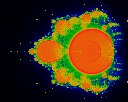Average age. That is, cycle count at which pixel was hit were accumulated, and then normalized by the measure at that pixel.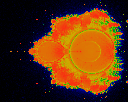Mean square deviation in the "age". Note how different details are highlighted.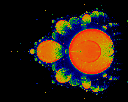Third moment of the "age". Further details not appearent in the measure or the Lyapunpov exponents become visible.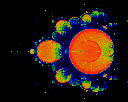Fourth moment of the "age". Further details not appearent in the measure or the Lyapunpov exponents become visible.Hausdorff measure, revisited. The iterated equation is still z = z*z +C, except that in this picture, we show the visited areas relative to C (i.e. we subtract C from z prior to plotting). Note that the circle (red dot) is centered about the origin. The origin is offset to show the large lobe to the right.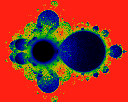Lyapunov exponenet. All non-black regions are replusive, with red representing the most strong repulsive regions.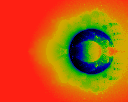Square distance between iterations; i.e (x-xlast)^2 + (y-ylast)^2 acumulated and normalized.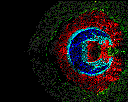Gaussian edge filter applied to above.Average orientation (angle) of jump, where a "jump" is the vector from the last to the current location. That is, phi = arctan ((y-ylast)/(x-xlast)) accumualted and normalized.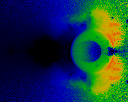Cosine of angle between current jump and last jump. The colormap used shows black = 0.0 (angle=90), red=1.0 (angle = 0). This is a very nice spirit-catcher, on it's side.A different colormap brings out different detail.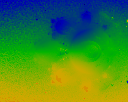Average X origin. The is, each pixel shows the average value of the real part of C where z = z2+C.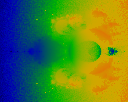Average Y origin. The is, each pixel shows the average value of the imaginary part of C where z = z2+C.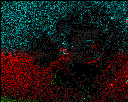Edge detection applied to average X origin.Edge detection applied to average Y origin.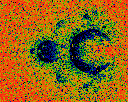Curl (dxY - dyX) of the average origin (X, Y). Curiously, the Divergence (dxX + dyY) looks qualitatively similar.Curl, as above, but with slightly different colormap.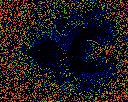Divergence. Note qualitative similarities, and subtle differences (which help reveal some of the minute artifacts in the other images).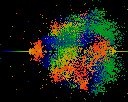Splat. Just a splat. Note the spiral structure, bottom center. How to clean this up ??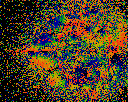Origins -- X. That is, each point is colored with the real part of C where z = z2 + C. Unlike the average origins image above, this is done with a "last point wins" coloring.Fine origins -- X. As above, but different colormap.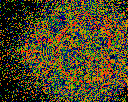Fine origins -- Y.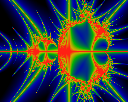Pickover's Stalks. Cliff Pickover hit on the novel concept of looking to see how closely the orbits of interior points come to the x and y axes. In these pictures, the closer that the point approaches, the higher up the color scale, with red denoting the closest approach. The logarithm of the distance is taken to accentuate the details.A closeup of the upper left area.How close do iterates come to the horizontal and vertical axes centered at (-0.25, 0.25) ?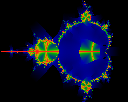How closely do iterates approach the cross, defined as (0==x, -0.2<y<0.2) + (-0.2<x<0.2, 0==y) ?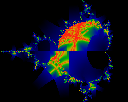How closely do iterates approach the cross centered at (-0.3, 0.2) ?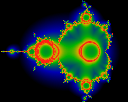Note that the previous images showed that some points inside every bud iterated quite close to the origin. Naively, it might seem that some point inside every bud iterates closely to some point inside the main cardiod. For example: How closely do iterates approach the circle, centered at the origin, of radius 0.2?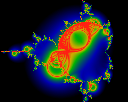How closely do iterates approach the circle, centered at (-0.3, 0.2), of radius 0.2?

The first image was created in 1988, as were variations on some of the others. All electronic copies were lost, with only polaroids and proof sheets surviving. New electronic copies were recreated in 1996 for this web exhibit.

Copyright (c) 1988, 1990, 1996, 1997 Linas Vepstas